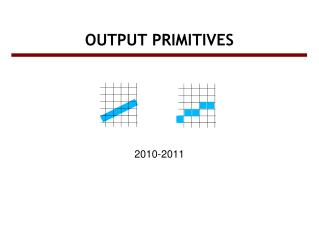Download PresentationOUTPUT PRIMITIVES# OUTPUT PRIMITIVES - PowerPoint PPT Presentation

Download Presentation##### OUTPUT PRIMITIVES

Download Policy: Content on the Website is provided to you AS IS for your information and personal use and may not be sold / licensed / shared on other websites without getting consent from its author. While downloading, if for some reason you are not able to download a presentation, the publisher may have deleted the file from their server.

- - - - - - - - - - - - - - - - - - - - - - - - - - - E N D - - - - - - - - - - - - - - - - - - - - - - - - - - -
##### Presentation Transcript

1. 2010-2011 OUTPUT PRIMITIVES

2. Screen vs. World coordinate systems • Objects positions are specified in a Cartesian coordinate system called World Coordinate System which can be three dimensional and real-valued. • Locations on a video monitor are referenced in integerscreen coordinates. Therefore object definitions has to be scan converted to discrete screen coordinate locations to be viewed on a video monitor.

3. Specification of a 2D WCS in OpenGL • glMatrixMode (GL_PROJECTION); glLoadIdentity (); gluOrtho2D (xmin, xmax, ymin, ymax); • Objects that are specified within these coordinate limits will be displayed within the OpenGL window.

4. Output Primitives • Graphic SW and HW provide subroutines to describe a scene in terms of basic geometric structures called output primitives. • Output primitives are combined to form complex structures • Simplest primitives • Point (pixel) • Line segment

5. Scan Conversion • Converting output primitives into frame buffer updates. Choose which pixels contain which intensity value. • Constraints • Straight lines should appear as a straight line • primitives should start and end accurately • Primitives should have a consistent brightness along their length • They should be drawn rapidly

6. OpenGL Point Functions • glBegin (GL_POINTS); glVertex2i(50, 100); glVertex2i(75, 150); glVertex2i(100, 200); glEnd();

7. OpenGL Line Functions • glBegin (GL_LINES); glVertex2iv(p1); glVertex2iv(p2); glVertex2iv(p3); glVertex2iv(p4); glVertex2iv(p5); glEnd(); p3 p5 p1 p4 p2

8. OpenGL Line Functions • glBegin (GL_LINE_STRIP); glVertex2iv(p1); glVertex2iv(p2); glVertex2iv(p3); glVertex2iv(p4); glVertex2iv(p5); glEnd(); p3 p5 p1 p4 p2

9. OpenGL Line Functions • glBegin (GL_LINE_LOOP); glVertex2iv(p1); glVertex2iv(p2); glVertex2iv(p3); glVertex2iv(p4); glVertex2iv(p5); glEnd(); p3 p5 p1 p4 p2

10. Line Drawing Algorithms • Simple approach:sample a line at discrete positions at one coordinate from start point to end point, calculate the other coordinate value from line equation (slope-intercept line equation).If m>1 , increment y and find xIf m≤1, increment x and find y Is this correct?

11. Digital Differential Analyzer • Simple approach: too many floating point operations and repeated calculations • Calculate from for a value

12. DDA • Is faster than directly implementing y=mx+b. No floating point multiplications. We have floating point additions only at each step. • But what about round-off errors? • Can we get rid of floating point operations completely?

13. Bresenham's Line Algorithm • DDA: Still floating point operations y=mx+b yk+1 dupper yk dlower xk xk+1 xk+2 xk+3

14. at step k+1: 0 if pk was negative 1 if pk was positive to calculate p0 at the starting pixel position (x0,y0)

15. Bresenham’s Line-Drawing Algorithm Input: two line end points (x0,y0) and (xend,yend) draw (x0,y0) pk←2Δy-Δx; xk←x0 while xk<xend xk+1←xk+1 if pk ≤ 0 choose yk yk+1←yk; pk+1←pk+2Δy elsechoose yk+1 yk+1←yk+1; pk+1←pk + 2Δy - 2Δx draw (xk+1,yk+1) xk←xk+1 pk←pk+1

16. Example from the textbook • Using Bresenham’s algorithm digitize the line with endpoints (20,10) and (30,18) Solution: • Δy = (18-10)=8, Δx = (30-20)=10, • m = Δy / Δx = 0.8, • p0= 2Δy - Δx = 16 – 10 = 6. • 2Δy = 16 and (2Δy) - 2Δx = 16 - 20 = -4. • plot the initial point (x0, y0) = (20,10 • find the following pixel positions along the line:

17. Example continued... 18 10 20 21 22 25 30

18. Plotted pixels 18 10 20 21 22 25 30